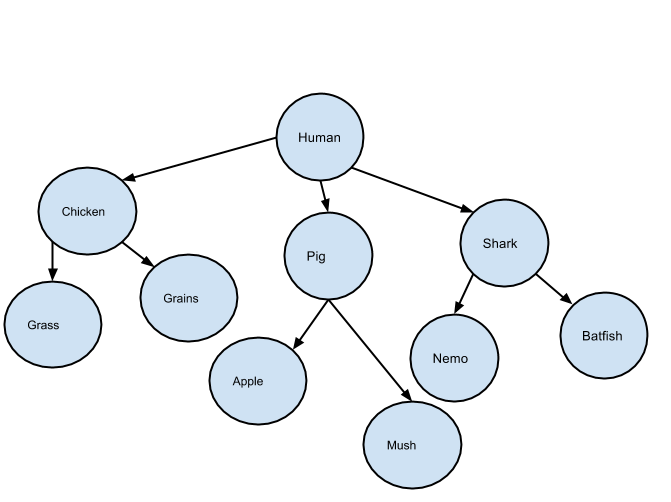# BFS and DFS with Python

GitHub

So I’ve found myself in a situation where I have had to revise simple Data Structures from my Software Engineering Degree. I have not done this for several years, so naturally my first step in this process was looking at the workings of various structures on Google (then subsequently Wikipedia). After refreshing my memory, I decided that the best thing to do was code up these algorithm.

In this blog post, I will show you the BFS (breadth first search) and the DFS (depth first search) in Python.

Unfortunately many of the already Python implementations of these algorithms on the web are either over complicated or have readability issues or don’t emphasise the difference between the two algorithms.

I will attempt to explain both, and give a readable and understandable explanation of both. Targeted towards first year Computer Science or Software Engineering students.
Just a small note before I move on. I remember doing this in first year University where we had used Java. It was a nightmare. I programmed this in Python today and it made me think how much more we could’ve learnt if we used Python at USyd… anyway, here goes!

If you know what stacks and queues are skip the below two paragraphs.

Queue A Queue is a computation abstraction of a real-word queue. So, imagine a queue at the movies. The first person to arrive will get served first, the second will get served second etc. Basically a queue is first-come-first-serve. In Computer Science terms, this would be first-in-first-out.

Stack A Stack is (guess what) just like a real-world Stack. So, just imagine a bunch of plates being washed. All of them are stacked up after the washing of the plates. Now someone hungry comes (probably me) and wishes to take a plate. He/she would take the first plate on the stack. So basically the last plate added on the stack of plates was the first one to be used. So a stack is, in computer science terms is, last-in-first-out.

A Graph
A graph is a structure where one object is able to be linked to one or more objects. An example of a graph is something like Facebook or a food chain. See below for an image from wikipedia:We call the ‘things’ nodes (in this case the numbers) and we call the links between two nodes the edges.

Now, let’s talk about the breadth first search. The BFS starts with any arbitrary node and makes it the current node, the BFS then checks if the current node is the node we are looking for. If it is the node we are looking for then search is completed. If it (the current node) is not then we take all the nodes that are linked to the current node and put them on a queue. We then take a node from the queue and it becomes the current node. The process is repeated until we find the node we need.DFS (Depth First Search)See what I did there? I simply copied the paragraph BFS (Breadth First Search) and made it the paragraph DFS (Depth First Search). By changing BFS with DFS and changing breadth with depth and changing queue with stack!

Implementation

Firstly, we need to learn how to represent a graph computationally. We can represent graphs as a list of lists. Below is an example of how the above graph is represented

[

``````1: \[2,5,9\],
2: \[3\],
3: \[4\],
5: \[6,8\],
6: \[7\],
9: \[10\]
``````

]

this basically tells us.

• 1 is connected to 2,5 and 9.
• 2 is connected to 3
• 3 is connected to 4
• 5 is connected to 6 and 8
• 6 is connected to 7
• 9 is connected to 10

In our example though. We will be using a dictionary of lists, because our keys are text. A dictionary basically converts text to a number anyway.

Below is the graph and the computational representation:

graph = {
“human” : [“chicken”,“pig”,“shark”],
“shark” : [“nemo”,“batfish”],
“batfish” : [“tuna”],

``````        "pig" : \["apple","mush"\],
"chicken" : \["grass","grains"\]
}
``````So how do we code DFS and BFS?

See below

def bfs(graph,root,whatToFind):
queue = Queue.Queue()
queue.put(root)
while queue:
curr = queue.get()
print curr
if whatToFind == curr:
print ‘found!’
return
if curr in graph:

def dfs(graph,root,whatToFind):
stack = []
stack.append(root)
while stack:
curr = stack.pop()
print curr
if whatToFind == curr:
print ‘found!’
return
if curr in graph: